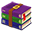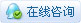1187浏览

# 7块钱可以做什么？用ATtiny13做一个非常酷的PoV显示器！5个ATtiny13单片机售价1.5美元。

ATtiny13对库的支持有限，所以除了让它点亮几个LED之外，我暂时没有想到其他玩法。PoV显示器基本上是一堆闪烁的LED，并有一些精心安排的延迟。而我发现ATtiny13和ATtiny85都有相同的引脚布局。

• 1 x ATtiny13
• 5个3毫米的LED（也可以用5毫米的LED，3毫米的看起来更好，因为可以更靠近PCB）
• 1个CR2032电池
• 1个CR2032电池座
• 1个滑动开关
• 原型PCB或定制PCB
• 电烙铁Arduino IDE相关设置https://mcudude.github.io/MicroCore/package_MCUdude_MicroCore_index.jsonok，现在你就可以从Arduino IDE中选择到ATtiny13了。

[td]
 Option Value Board ATtiny13 BOD 2.7v Clock 9.6Mhz internal Osc. Timing "Micors Disabled" Port Select Serial Port in which your Arduino is connected Programmer Arduino as ISP (MicroCore)[td]
 ATtiny13 Pin Arduino Pin 1 10 5 11 6 12 7 13 8 5v 4 Ground (GND)``````// https://github.com/B45i/Tiny-PoV
// App to calculate array values: https://pov-display-calc.vercel.app/
// Preact app source: https://github.com/B45i/pov-display-calc

#include <avr/pgmspace.h>

#define DELAY_TIME 1
#define CHAR_BREAK 2

uint8_t leds[] = { 0, 1, 2, 3, 4 };
uint8_t keys[] = { 1, 2, 4, 8, 16 };

void setup() {
for (uint8_t i = 0; i < 5; i++) {
pinMode(leds[i], OUTPUT);
}
}

const PROGMEM uint8_t alphabets[] = {
{ 0, 0, 0, 0, 0 },       // Space
{ 30, 5, 5, 30, 0 },     // A
{ 31, 21, 21, 10, 0 },   // B
{ 14, 17, 17, 10, 0 },   // C
{ 31, 17, 17, 14, 0 },   // D
{ 31, 21, 21, 17, 0 },   // E
{ 31, 20, 20, 16, 0 },   // F
{ 14, 17, 19, 10, 0 },   // G
{ 31, 4, 4, 4, 31 },     // H
{ 0, 17, 31, 17, 0 },    // I
{ 0, 17, 30, 16, 0 },    // J
{ 31, 4, 10, 17, 0 },    // K
{ 31, 1, 1, 1, 0 },      // L
{ 31, 12, 3, 12, 31 },   // M
{ 31, 12, 3, 31, 0 },    // N
{ 14, 17, 17, 14, 0 },   // O
{ 31, 20, 20, 8, 0 },    // P
{ 14, 17, 19, 14, 2 },   // Q
{ 31, 20, 22, 9, 0 },    // R
{ 8, 21, 21, 2, 0 },     // S
{ 16, 16, 31, 16, 16 },  // T
{ 30, 1, 1, 30, 0 },     // U
{ 24, 6, 1, 6, 24 },     // V
{ 28, 3, 12, 3, 28 },    // W
{ 17, 10, 4, 10, 17 },   // X
{ 17, 10, 4, 8, 16 },    // Y
{ 19, 21, 21, 25, 0 },   // Z
{ 31, 17, 31, 0, 0 },    // 0
{ 18, 31, 16, 0, 0 },    // 1
{ 29, 21, 23, 0, 0 },    // 2
{ 21, 21, 31, 0, 0 },    // 3
{ 7, 4, 31, 4, 0 },      // 4
{ 23, 21, 29, 0, 0 },    // 5
{ 31, 21, 29, 0, 0 },    // 6
{ 1, 1, 31, 0, 0 },      // 7
{ 31, 21, 31, 0, 0 },    // 8
{ 23, 21, 31, 0, 0 },    // 9
};

void displayLine(uint8_t line) {
for (uint8_t i = 0; i < 5; i++) {
digitalWrite(leds[i], (line & keys[i]) == keys[i]);
}
}

void displayLetter(uint8_t n) {
for (uint8_t i = 0; i < 5; i++) {
delay(DELAY_TIME);
}
displayLine(0);
}

void displayString(char *s) {
for (uint8_t i = 0; i < strlen(s); i++) {
uint8_t index;

if (s[i] == ' ') {
index = 0;
} else if (isalpha(s[i])) {
index = (uint8_t)toupper(s[i]) - 64;
} else if (isdigit(s[i])) {
index = (uint8_t)(s[i]) - 21;
}

displayLetter(index);
delay(CHAR_BREAK);
}
}

void loop() {
displayString("HELLO 123 ");
}复制代码``````

``displayString("HELLO 123 "); // 替换成你想要显示的文本复制代码``

``````  int a[] = {
{0, 1, 1, 0, 0},
{1, 0, 0, 1, 0},
{1, 1, 1, 1, 0},
{1, 0, 0, 1, 0},
{1, 0, 0, 1, 0},
};

for (int i = 0; i < 5; i++) {
for (int j = 0; j < 5; j++) {
digitalWrite(LEDs[j], a[j][i]);
}
}复制代码``````

PoV显示器有5行和5列，所以我们可以使用一个长度为5的数组。

[td]
 LED Enum LED 1 2^0= 1 LED 2 2^1= 2 LED 3 2^2= 4 LED 4 2^3= 8 LED 5 2^4= 16[td]
 列 值 第1列 0 + 2 + 4 + 8 + 16 = 30 第2列 1 + 0 + 4 + 0 + 0 = 5 第3列 1 + 0 + 4 + 0 + 0 = 5 第4列 0 + 2 + 4 + 8 + 16 = 30 第5列 0 + 0 + 0 + 0 + 0 = 0 (所有LED全不亮)[td]
 运算 结果 LED状态 30 & 1 == 1 false 关闭LED1 30 & 2 == 2 true 点亮LED2 30 & 4 == 4 true 点亮LED3 30 & 4 == 8 true 点亮LED4 30 & 16 == 16 true 点亮LED530二进制是11110PoV显示电路是相当简单的，可以用下面的电路图来连接电路。PCB文件可以在文末下载。3 MB, 下载次数: 2739.55 KB, 下载次数: 15

 赞

 本帖最后由 诩 于 2022-5-13 08:34 编辑 确定只要七块钱？

 诩 发表于 2022-5-13 08:33 确定只要七块钱？ 我怕一会儿整太多钱了我妈会问候我您需要登录后才可以回帖 登录 | 立即注册 本版积分规则 回帖并转播 回帖后跳转到最后一页